# Forecasting Daily New Confirmed COVID-19 Cases in Maldives — Part 1

Predicting Daily New Cases using Simple Exponential Smoothing, Linear Trend Model, and Holt-Winters Smoothing.Photo by Fusion Medical Animation on Unsplash

This first article will explain how to forecast daily new COVID-19 confirmed cases in Maldives using three different models. Check out my second article about forecasting using ARIMA models here.

# Introduction

Many parties have widely discussed the development of COVID-19 from 2020 to this year, especially online media. Through digital platforms such as social media, news, or online articles, news about the development of COVID-19 spread continues to be reported every day. Furthermore, with the vaccine that has begun to be distributed to the public, several media, institutions, and researchers from various countries have begun to predict or forecast the development of future COVID-19 cases in their country or other countries by using various forecasting techniques/methods, to be able to predict the development of new COVID-19 cases.

In this article, forecasting the number of cases will be carried out to predict daily new confirmed COVID-19 cases in the Maldives country using several forecasting methods and models. The data used in this article takes data from Our World in Data (2021) from January 1, 2021 to November 6, 2021.

# Time Series Components and Types

Before exploring time series plot of daily new cases in Maldives, let’s understand the components of time series. There are 4 components, namely:

1. Trend: The trend indicates the data’s overall tendency to increase or decrease over time. A trend is a long-term, continuous, broad-based average tendency. The increases or decreases do not need to be consistent across a specific period. Examples of the trend are increased pollution, increased demand for eggs, or increased literacy rate
2. Seasonal Variation: Seasonal variations are any periodic variation of less than a year’s duration. Examples of seasonal variation are increased sales of coffee in the winter, increased sales of ice cream in the summer, increased prices of various fruits and vegetables.
3. Cyclical Variation: Cyclical variance is a non-seasonal component that fluctuates in a predictable cycle. An example of cyclical variation is that financial indicators are impacted by business cycles of around 5 to 7 years.
4. Irregular/Random Variation: The variable under investigation is influenced by a factor whose structure is wholly random or irregular. The examples of random variation are the spike in steel prices due to a manufacturing strike, flood, quickening of earth, or war.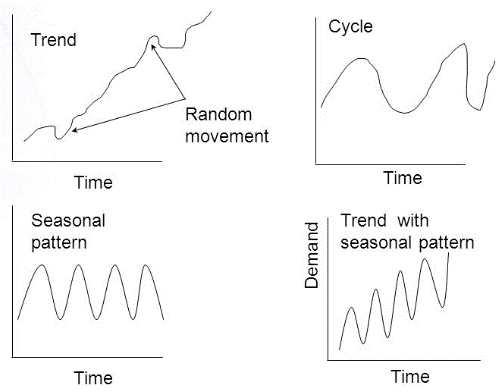Time Series Component by Ullah (2020)

In addition, according to Smigel (2021), there are two types of time series. The first one is stationary time series, which means that if the mean, variance, and autocorrelation remain constant across the period. The second one is non-stationary time series, which means that if the mean, variance, and autocorrelation change across the period.

# Maldives New Cases Time Series Plot

After knowing all the time series components, the time series plot for daily new confirmed COVID-19 cases in the Maldives will be created in this section. The following is a time series plot that has been made: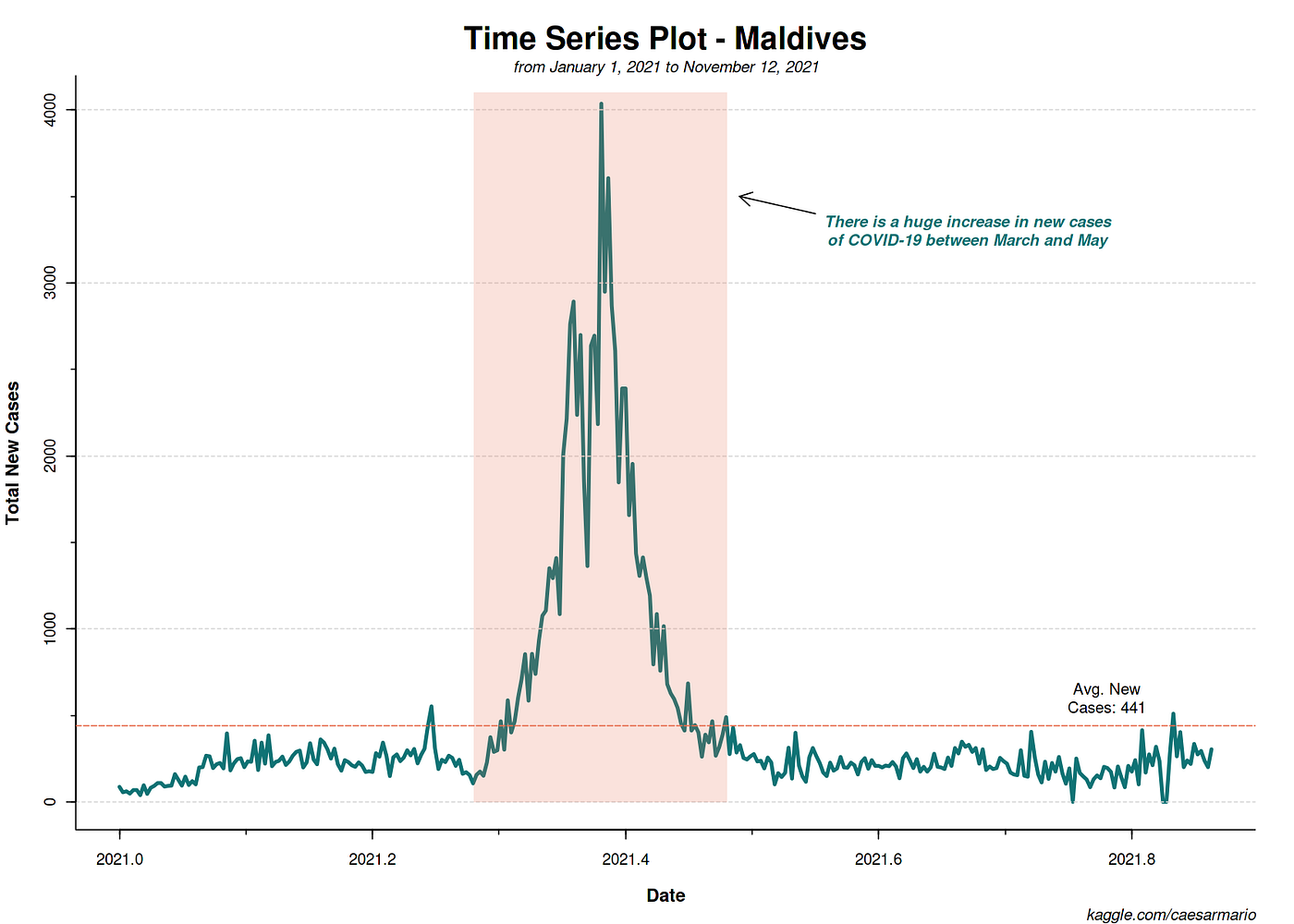Time Series Plot of New COVID-19 Cases in Maldives

It can be seen that this time series plot is non-stationary since the means, variance, and covariance in this time series plot constantly change due to unpredictable upward and downward movements. In addition, there are irregular variations in this time series plot, where unexpected movements or fluctuations occur, random variations, and occur in a short time. Furthermore, there are no cyclical variations, trends, and seasonal components in this time series plot since the upward and downward movements are not repeated. Also, the dataset used is a daily dataset and less than a year (from early January 2021 to early November 2021).

In order to prove that this time series plot is non-stationary, several statistical tests will be carried out. Statistical tests that will be carried out are the Mann-Kendall trend test, autocorrelation function (ACF) plot, unit root test, and non-statistical test using the ‘ndiffs’ and ‘nsdiffs’ function.

## Mann-Kendall Trend Test

The Mann-Kendall Test is a statistical test that detects if the time series is monotonously increasing or decreasing in trend (Zaiontz, 2021). The following is the hypothesis for the Mann-Kendall trend test.

* Hypothesis:

H0: There is no trend available in the series.

H1: There is a trend available in the series.

* Criteria:

If the p-value is < 0.05, reject H0.

It can be seen that the p-value is 0.6144, which is greater than 0.05. It can be concluded that there is no trend component available in the series (H0 accepted).

## Autocorrelation Function (ACF) Plot

An ACF Plot (referred to as a correlogram or autocorrelation diagram) is a plot used to illustrate serial correlation in time series data (Glen, 2016). Lags are the time intervals between those preceding times (Lee, 2021).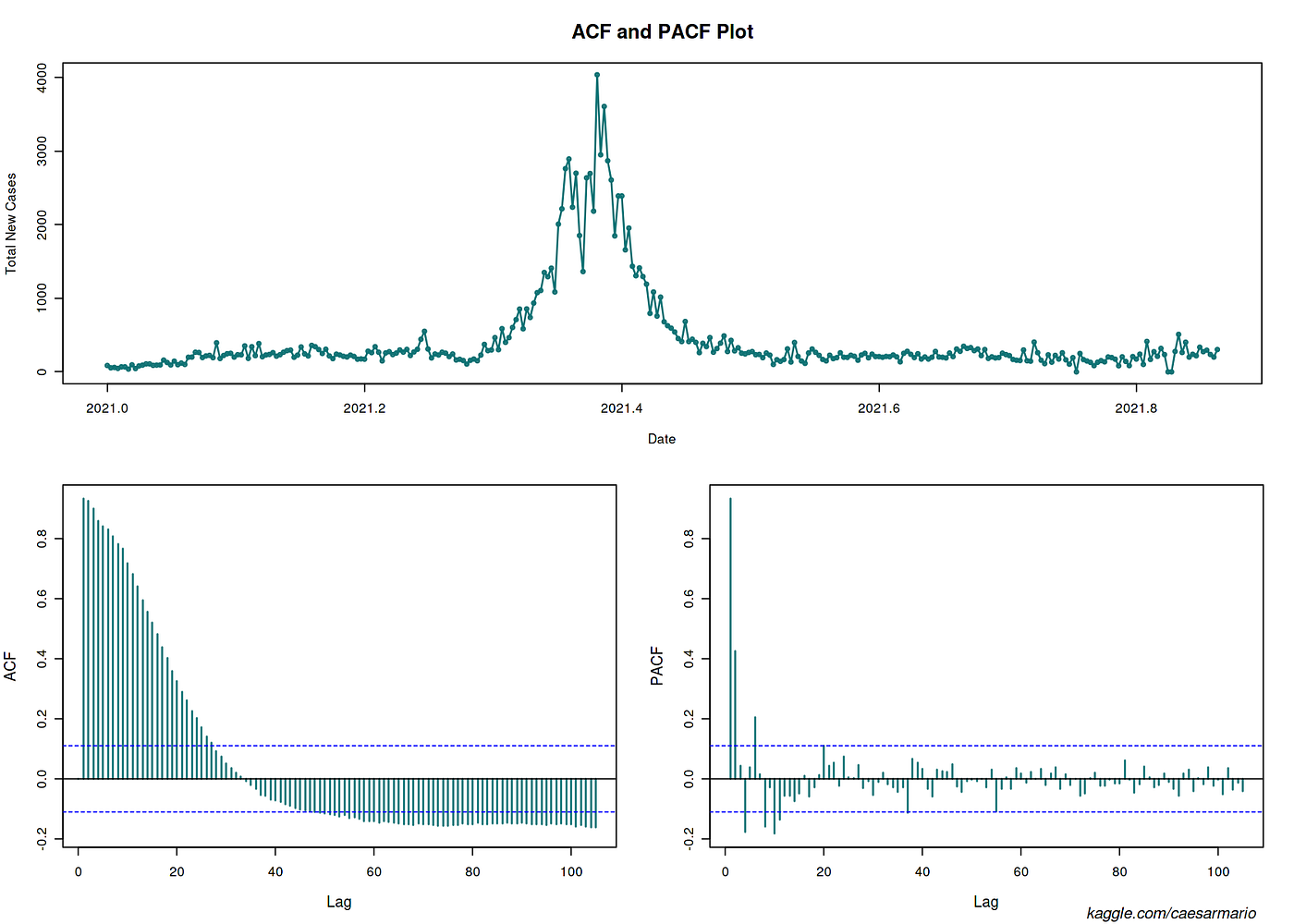ACF & PACF Plot of Time Series

From the ACF plot above, it can be concluded that the series is not stationary because autocorrelation remains significant for the first several (27) lags and the ACF plot dies down exceptionally slowly.

## Unit Root Test

A unit root test is used to analyze and determine if a time series is stationary (Verma, 2021). In this section, the unit root tests used are the Augmented Dickey-Fuller (ADF) test and the Kwiatkowski–Phillips–Schmidt–Shin (KPSS) test.

> Augmented Dickey-Fuller test

* Hypothesis:

H0: The series is not stationary.

H1: The series is stationary.

* Criteria:

If the p-value is < 0.05, reject H0.

Based on the results of the ADF test, the p-value is 0.673, which is greater than 0.05. It can be concluded that the series is not stationary (H0 accepted).

> Kwiatkowski–Phillips–Schmidt–Shin test

* Hypothesis:

• H0: The series is stationary.

• H1: The series is not stationary.

* Criteria:

• If the p-value is < 0.05, reject H0.

• If the value of the test-statistic is greater than the critical value, reject H0.

Based on the results of the KPSS test, the p-value is 0.03264, which is smaller than 0.05. In addition, the test-statistic value is more significant than the critical value (0.5401 greater than 0.463). From these results, it can be concluded that the series is not stationary (H0 accepted).

## Non-Statistical Test

The non-statistical test in this section will use the ‘ndiffs’ and ‘nsdiffs’ function, where the ‘ndiffs’ function is used to determine the number of differencing needed to get the stationary time series, and the ‘nsdiffs’ is used to determine the number of seasonal differencing needed.

It can be seen that the number of differencing required to obtain a stationary time series is 1. Based on these results, it can be concluded that the time series plot before differencing is non-stationary. In addition, it can be seen that the number of seasonal differencing needed is 0, which means that seasonal differencing is not needed for this time-series data because this time series data does not have seasonality.

## Analysis Conclusion

From the statistical and non-statistical test results, it can be concluded that it is true that the time series is non-stationary. In addition, based on the Mann-Kendall trend test and ACF plot, it can be concluded that this time series does not have a trend and seasonality component.

# Forecasting Techniques

In this section, forecasting techniques (SES, linear trend model, and Holt-Winters smoothing) will be used to forecast daily new COVID-19 cases in Maldives. But first, data partitioning will be performed before using any forecasting approach to overcome the overfitting problem.

The data partitioning ratio is 80:20, where 80% of the data set is as training, and the rest (20%) is testing.

After partitioning, it can be seen that the dataset has been divided into train and test with a training size of 253 data, while the test is 63 data.

## Simple Exponential Smoothing

SES is a practical and straightforward forecasting approach that uses an exponentially weighted average of earlier data to get the prediction. In SES, the time series data assigns declining weights to recent data and less to older data. SES is used to forecast time series when the data lacks trend and seasonality. SES is also limited to estimating the level component. Each parameter’s weight, or weight reduction, is always defined by a smoothing parameter called α (alpha), which values between 0 and 1.

• α = 0, indicating that future forecasted values are based on the average of past data.
• α = 1, indicating that future forecasted values are based on current observation.

It can be seen that the SES model can have good training accuracy and the forecast results on the graph are also good. However, the forecast values generated for the SES model remain constant, which is 194.8338.

## Linear Trend Model

Linear trend model is a subset of the basic regression model, with time t as the independent variable. It is applied in time series where the mean increases smoothly, indicating a consistent trend. The linear trend model determines the slope and intercepts that best suit the historical data.

It can be seen that the linear trend model has a reasonably poor training accuracy and the forecast results on the graph/values are also insufficient. The p-value of the linear trend model is 0.138, which indicates that there is no trend component (p-value generated > 0.05).

## Holt-Winters Smoothing

Holt-Winters is a forecasting model that includes three characteristics of time series: average, trend, and seasonality. Holt-Winters technique is a modification of the Holt exponential smoothing technique that enables it to be utilized in both trend and seasonality. Holt-Winters method transforms three different smoothing techniques: SES, Holt’s exponential smoothing (HES), and winter’s exponential smoothing (WES). In Holt-Winters, there are two methods, namely additive and multiplicative.

It can be seen that the training accuracy of the Holt-Winters model is quite good. However, the forecast plot and values for Holt-Winters show a decrease in each period until it reaches a negative number.

## Model Comparison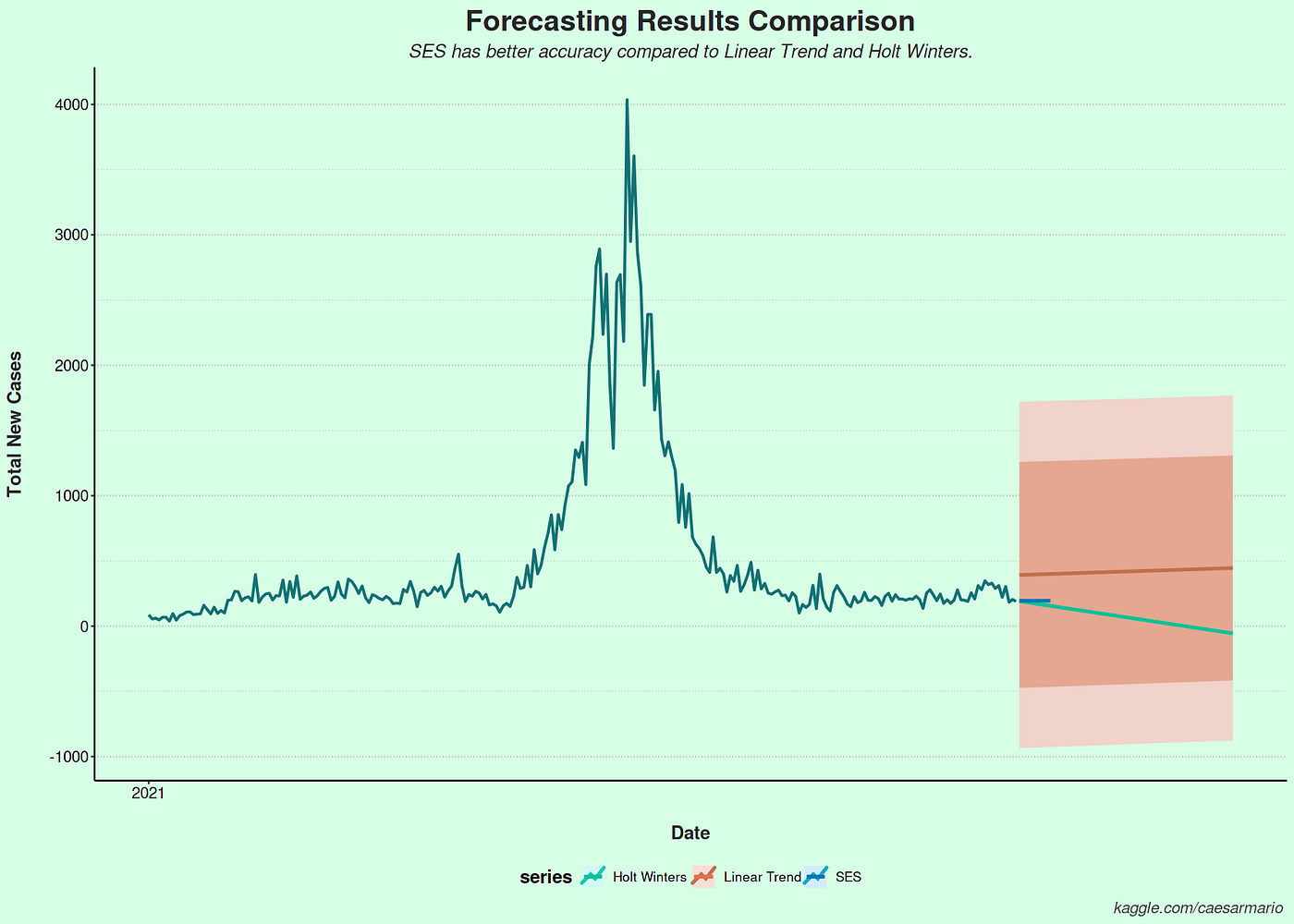Comparison of SES, Linear Trend, and Holt-Winters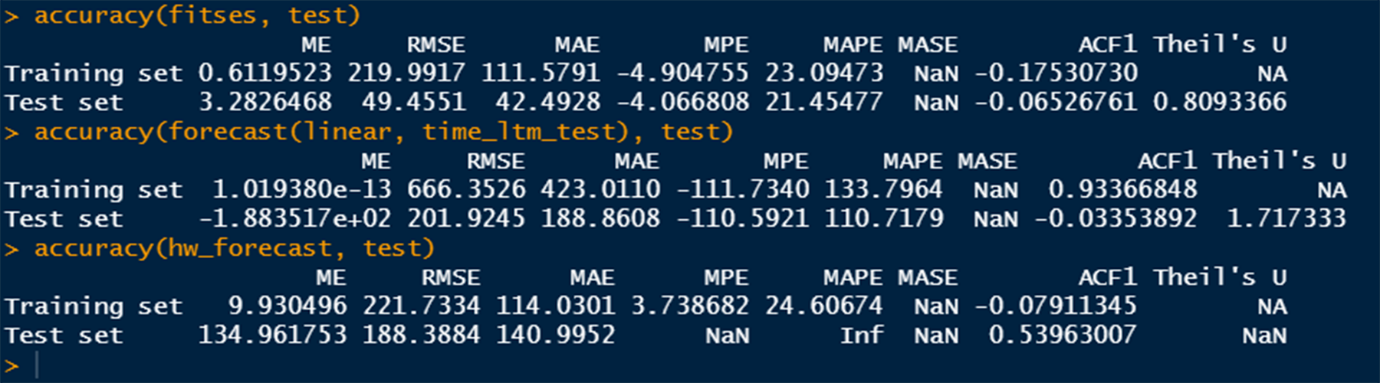Performance Results of SES, Linear Trend, and Holt-Winters

From the plot, ot can be seen that the SES model has the best accuracy. However, based on accuracy, it can be seen that all the models are underfitting. The RMSE and MAE train and test results produced by the SES model are better compared to other two models.

From MAPE results, the SES model can provide an error percentage of 23% for training and 21% for testing, while linear trend model MAPE value is up to 110% for test and 133% for training. MAPE value for Holt-Winters is slightly higher than SES (24.6%).

Theil’s U result shows that the SES model also fits significantly than the Naïve forecast since it is lower than 1. The linear trend model Theil’s U results are higher than 1, indicating that this model fits poorly than the Naïve forecast.

This first article will explain how to forecast daily new COVID-19 confirmed cases in Maldives using three different models. Check out my second article about forecasting using ARIMA models here.

All codes are available here

--

--